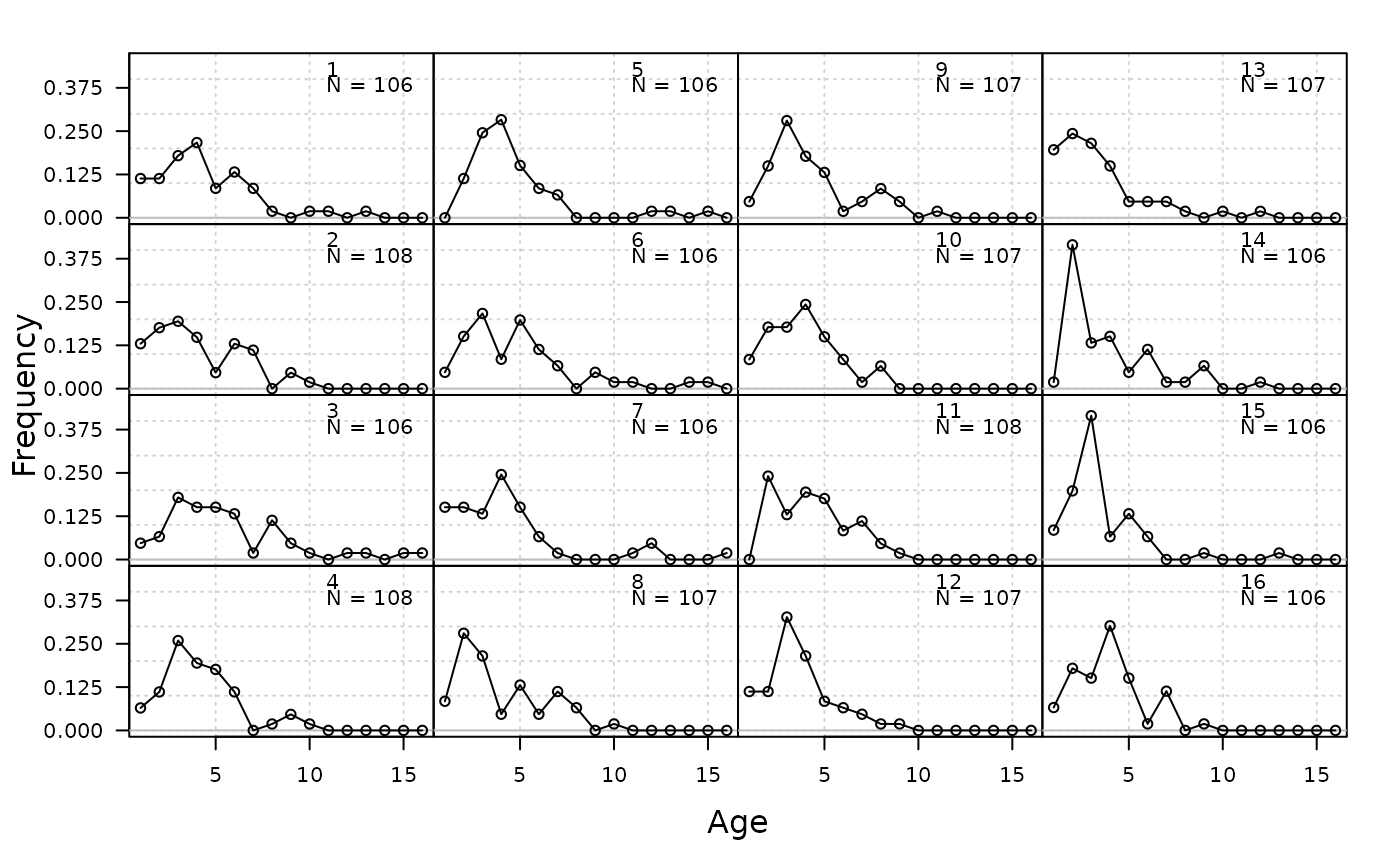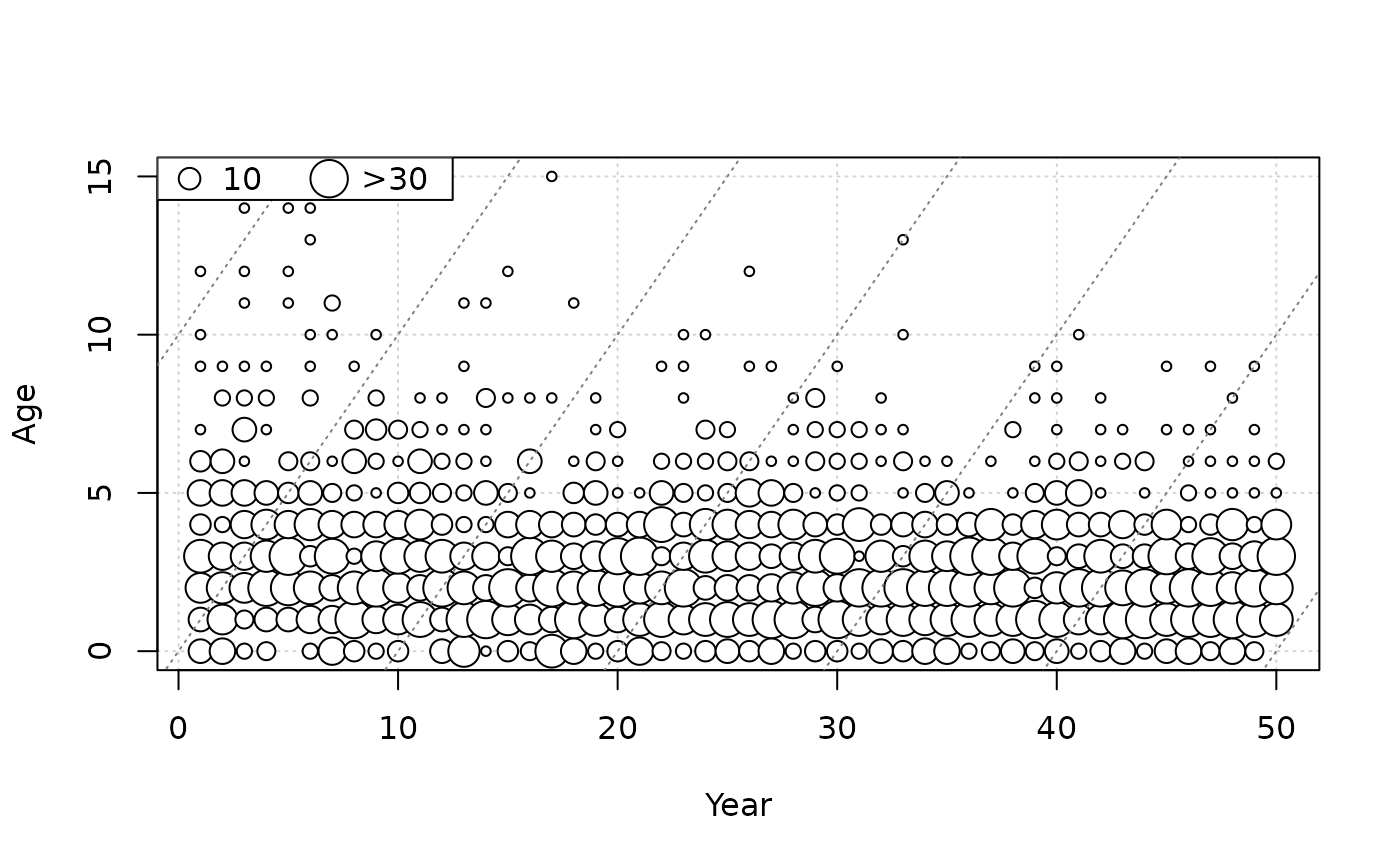Plots annual length or age composition data.

plot_composition(
Year = 1:nrow(obs),
obs,
fit = NULL,
plot_type = c("annual", "bubble_data", "bubble_residuals", "mean"),
N = rowSums(obs),
CAL_bins = NULL,
ages = NULL,
ind = 1:nrow(obs),
annual_ylab = "Frequency",
annual_yscale = c("proportions", "raw"),
bubble_color = c("black", "white"),
fit_linewidth = 3,
fit_color = "red"
)

## Arguments

Year

A vector of years.

obs

A matrix of either length or age composition data. For lengths, rows and columns should index years and length bin, respectively. For ages, rows and columns should index years and age, respectively.

fit

A matrix of predicted length or age composition from an assessment model. Same dimensions as obs.

plot_type

Indicates which plots to create. Options include annual distributions, bubble plot of the data, and bubble plot of the residuals, and annual means.

N

Annual sample sizes. Vector of length nrow(obs).

CAL_bins

A vector of lengths corresponding to the columns in obs. and fit. Ignored for age data.

ages

An optional vector of ages corresponding to the columns in obs.

ind

A numeric vector for plotting a subset of rows (which indexes year) of obs and fit.

annual_ylab

Character string for y-axis label when plot_type = "annual".

annual_yscale

For annual composition plots (plot_type = "annual"), whether the raw values ("raw") or frequencies ("proportions") are plotted.

Numeric, for adjusting the relative size of bubbles in bubble plots (larger number = larger bubbles).

bubble_color

Colors for negative and positive residuals, respectively, for bubble plots.

fit_linewidth

Argument lwd for fitted line.

fit_color

Color of fitted line.

## Value

Plots depending on plot_type.

Q. Huynh

## Examples

plot_composition(obs = SimulatedData@CAA[1, 1:16, ])plot_composition(obs = SimulatedData@CAA[1, , ], plot_type = "bubble_data",
ages = 0:SimulatedData@MaxAge)